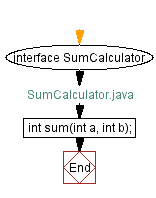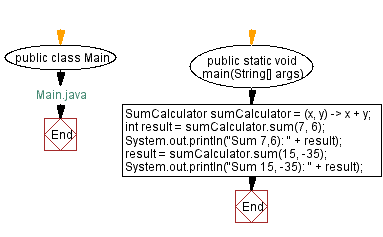# Java Lambda expression - Sum of two integers

## Java Lambda Program: Exercise-1 with Solution

Write a Java program to implement a lambda expression to find the sum of two integers.

Sample Solution:

Java Code:

``````// SumCalculator.java

interface SumCalculator {
int sum(int a, int b);
}
```
```
``````// Main.java
public class Main {
public static void main(String[] args) {
SumCalculator sumCalculator = (x, y) -> x + y;
int result = sumCalculator.sum(7, 6);
System.out.println("Sum 7,6): " + result);
result = sumCalculator.sum(15, -35);
System.out.println("Sum 15, -35): " + result);
}
}
``````

Sample Output:

```Sum 7,6): 13
Sum 15, -35): -20
```

Explanation:

In the above exercise, a functional interface named SumCalculator is defined. This interface has a single abstract method called sum that takes two integer parameters and returns an integer result. The lambda expression (x, y) -> x + y is used to implement this method. It takes two integers, x and y, and returns their sum.

In the main method, an instance of the SumCalculator interface is created using the lambda expression and assigned to the variable sumCalculator. For calculating the sum, the sumCalculator is invoked with pairs of integer arguments. System.out.println prints the results to the console from the result variable.

Flowchart:Live Demo:

Java Code Editor:

Improve this sample solution and post your code through Disqus

Java Lambda Exercises Previous: Java Lambda expression Exercises Home.
Java Lambda Exercises Next: Check if string is empty.

What is the difficulty level of this exercise?

Test your Programming skills with w3resource's quiz.

﻿

## Java: Tips of the Day

DropElements

Removes elements in an array until the passed function returns true. Returns the remaining elements in the array.

Loop through the array, using Arrays.copyOfRange() to drop the first element of the array until the returned value from the function is true. Returns the remaining elements.

```public static int[] dropElements(int[] elements, IntPredicate condition) {
while (elements.length > 0 && !condition.test(elements)) {
elements = Arrays.copyOfRange(elements, 1, elements.length);
}
return elements;
}
```

Ref: https://bit.ly/37YWqzD

We are closing our Disqus commenting system for some maintenanace issues. You may write to us at reach[at]yahoo[dot]com or visit us at Facebook